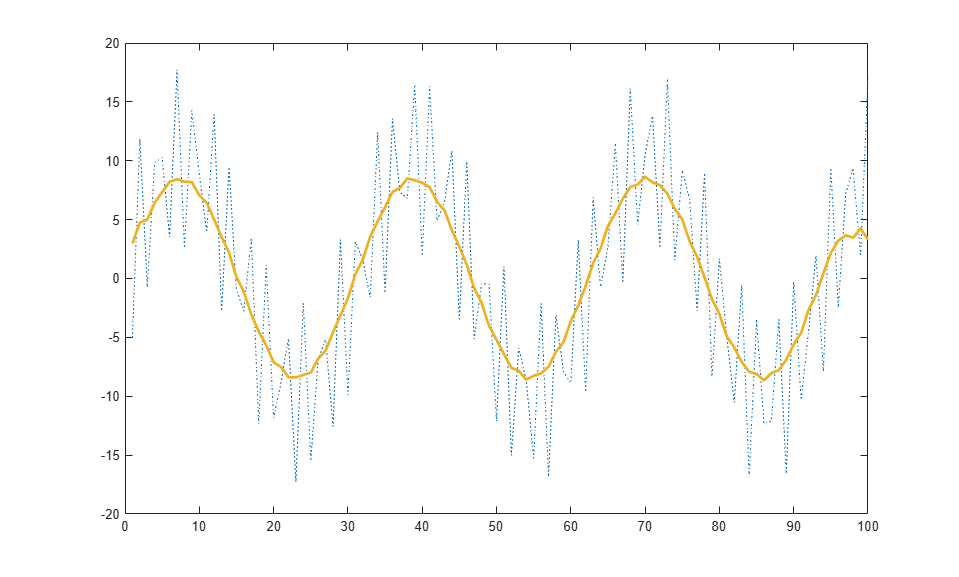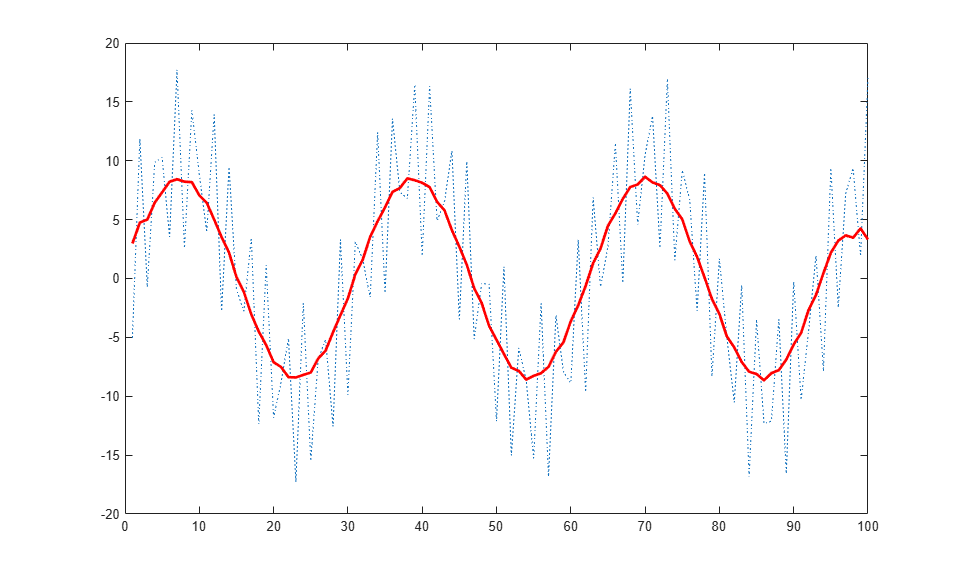# update

Class: matlab.graphics.chartcontainer.ChartContainer
Package: matlab.graphics.chartcontainer

Update instance of chart container subclass after setting properties

## Syntax

```update(obj) ```

## Description

`update(obj)` updates the contents of the chart after one or more public property values change. Define this method to update the underlying graphics objects in the chart using the new property values. This method executes during the next `drawnow` execution, after the user changes one or more property values on the chart.

## Input Arguments

expand all

Object of the class that inherits from the `matlab.graphics.chartcontainer.ChartContainer` base class.

## Attributes

 `Abstract` `true` `Protected` `true`

To learn about attributes of methods, see Method Attributes.

## Examples

expand all

Define a class called `SmoothPlot` that plots a set of data using a dotted blue line with a smoothed version of the line.

To define the class, create a file called `SmoothPlot.m` that contains the following class definition with these features:

• `XData` and `YData` public properties that store the x- and y-coordinate data for the original line.

• `SmoothColor` and `SmoothWidth` public properties that control the color and width of the smooth line.

• `OriginalLine` and `SmoothLine` private properties that store the `Line` objects for original and smoothed data.

• A `setup` method that initializes `OriginalLine` and `SmoothLine`.

• An `update` method that updates the plot when the user changes the value of a property.

• A `createSmoothData` method that calculates a smoothed version of `YData`.

```classdef SmoothPlot < matlab.graphics.chartcontainer.ChartContainer properties XData (1,:) double = NaN YData (1,:) double = NaN SmoothColor (1,3) double {mustBeGreaterThanOrEqual(SmoothColor,0),... mustBeLessThanOrEqual(SmoothColor,1)} = [0.9290 0.6940 0.1250] SmoothWidth (1,1) double = 2 end properties(Access = private,Transient,NonCopyable) OriginalLine (1,1) matlab.graphics.chart.primitive.Line SmoothLine (1,1) matlab.graphics.chart.primitive.Line end methods(Access = protected) function setup(obj) % Create the axes ax = getAxes(obj); % Create the original and smooth lines obj.OriginalLine = plot(ax,NaN,NaN,'LineStyle',':'); hold(ax,'on') obj.SmoothLine = plot(ax,NaN,NaN); hold(ax,'off') end function update(obj) % Update line data obj.OriginalLine.XData = obj.XData; obj.OriginalLine.YData = obj.YData; obj.SmoothLine.XData = obj.XData; obj.SmoothLine.YData = createSmoothData(obj); % Update line color and width obj.SmoothLine.Color = obj.SmoothColor; obj.SmoothLine.LineWidth = obj.SmoothWidth; end function sm = createSmoothData(obj) % Calculate smoothed data v = ones(1,10)*0.1; sm = conv(obj.YData,v,'same'); end end end ```

Next, create a pair of `x` and `y` vectors. Plot `x` and `y` by calling the `SmoothPlot` constructor method, which is provided by the `ChartContainer` class. Specify the `'XData'` and `'YData'` name-value pair arguments and return the object as `c`.

```x = 1:1:100; y = 10*sin(x./5) + 8*sin(10.*x + 0.5); c = SmoothPlot('XData',x,'YData',y); ```Use `c` to change the color of the smooth line to red.

```c.SmoothColor = [1 0 0]; ```